/
/
/
101.Assume that the graphs show a competitive market for the
Not my Question
Flag Content

# Question : 101.Assume that the graphs show a competitive market for the : 1321642

101.Assume that the graphs show a competitive market for the product stated in the question.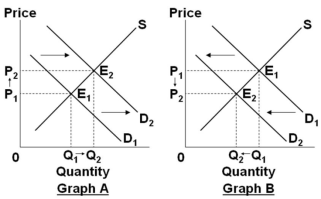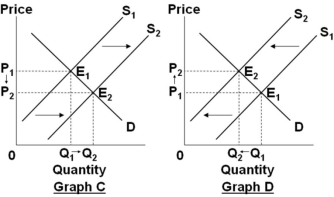Select the graph above that best shows the change in the market specified in the following situation: In the market for chicken, when the price of a substitute, such as beef, decreases.

A. Graph A

B. Graph B

C. Graph C

D. Graph D

102.Assume that the graphs show a competitive market for the product stated in the question.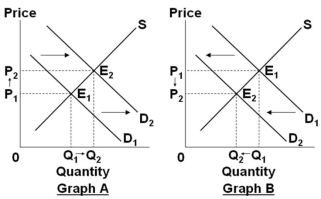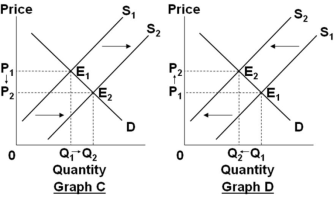Select the graph above that best shows the change in the market specified in the following situation: In the market for digital cameras, when the productivity of workers in the digital camera industry increases.

A. Graph A

B. Graph B

C. Graph C

D. Graph D

103.Assume that the graphs show a competitive market for the product stated in the question.Select the graph above that best shows the change in the market specified in the following situation: In the market for houses, when consumers experience a substantial fall in income due to a serious economic recession.

A. Graph A

B. Graph B

C. Graph C

D. Graph D

104.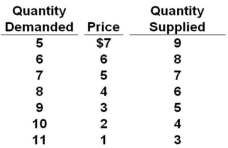Refer to the above table. If demand decreased by 4 units at each price, what would the new equilibrium price and quantity be?

A. \$3 and 5 units

B. \$4 and 6 units

C. \$5 and 7 units

D. \$6 and 8 units

105.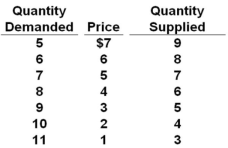Refer to the above table. If supply decreased by 2 units at each price, what would the new equilibrium price and quantity be?

A. \$3 and 5 units

B. \$4 and 4 units

C. \$5 and 5 units

D. \$6 and 6 units

106.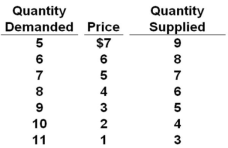Refer to the above table. If demand decreased by 4 units at each price and supply decreased by 2 units at each price, what would the new equilibrium price and quantity be?

A. \$3 and 5 units

B. \$4 and 4 units

C. \$5 and 5 units

D. \$6 and 6 units

107.The following data show the supply and demand schedules for a product.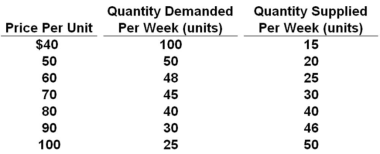Refer to the above data. The government now introduces a subsidy payment to producers of \$30 per unit. Assuming a purely competitive market for the product, the new equilibrium price will be between:

A. \$40-\$50

B. \$50-\$60

C. \$60-\$70

D. \$70-\$80

108.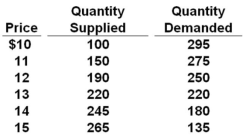Refer to the above table. If a technological advance lowers production costs such that the quantity supplied increases by 60 units of this product at each price, the new equilibrium price would be:

A. \$11

B. \$12

C. \$13

D. \$14

109.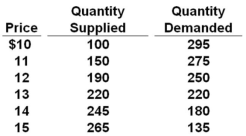Refer to the above table. A technological advance lowers production costs such that the quantity supplied increases by 60 units of this product at each price. As a result of this technological change, equilibrium output in this market:

A. Decreased by 60 units

B. Increased by 60 units

C. Increased by 30 units

D. Decreased by 30 units

110.A and B are substitute goods, but A and C are complementary goods. If the costs of producing A decreases, then the demand for:

A. Both B and C will decrease

B. Both B and C will increase

C. B will increase and the demand for C will decrease

D. B will decrease and the demand for C will increase

## Solution 5 (1 Ratings )

Solved
Finance 10 Months Ago 62 Views